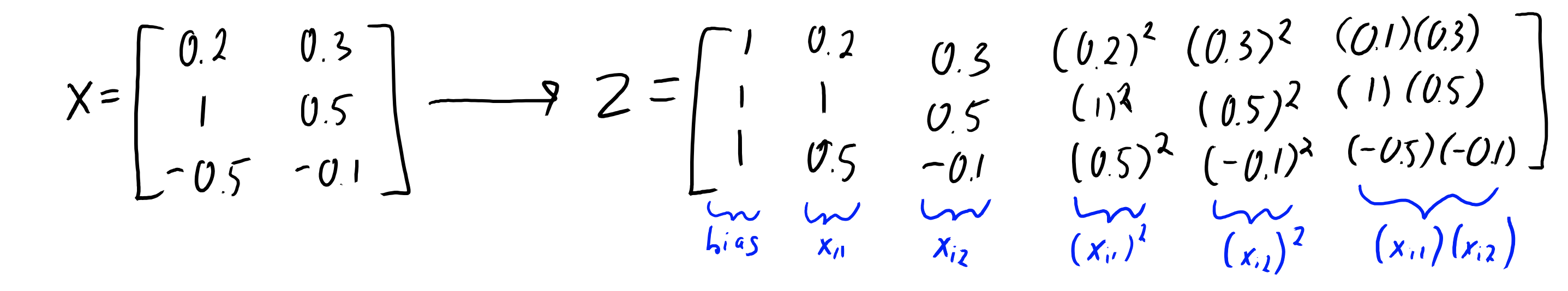Effectively by constructing new features that take the variable to certain powers. To get a y-intercept (bias), we just raise to the 0th power to get 1. We can fit polynomials of degree by raising other powers:

As the polynomial degree increases, the training error goes down but the approximation error goes up.

Choosing a basis is hard! We can do something like Gaussian radial basis functions (RBFs) or polynomial basis as these are both universal approximators given enough data.

## Kernel Trick §

Let be the basis. With multi-dimensional polynomial bases, actually forming which is is intractable.

Represent each column of as a unique term. For example, with an of , we can use to getWe compute

and for testing:

The key idea behind “kernel trick” for certain bases (like polynomials) is that we can efficiently compute and even though forming and is intractable.

We call the Gram Matrix.

Finally, we call the general degree-p polynomial kernel function . Computing is only time instead of .

Thus, computing is :

1. Forming takes time
2. Inverting which is a takes

All of our distance-based methods have kernel versions

## Learned Basis §

We can also learn basis from data as well. See latent-factor model